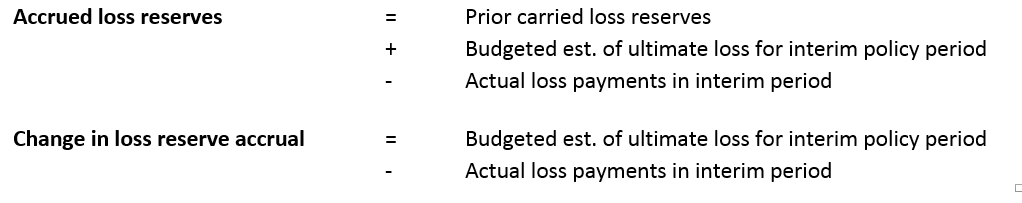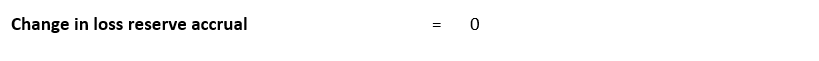# The Financial Implications of an Actuarial Reserve Report (for Self-Insureds)Business team analyzing charts and graphs

This article examines the financial implications of changes in actuarial loss reserve estimates for self-insured organizations.

An actuarial loss reserve analysis has the potential to affect an organization’s financial position. This potential is formally recognized by an organization when the liabilities on its balance sheet are adjusted to equal the actuarial reserve recommendations. The magnitude of the required balance sheet adjustment will depend, in part, on the type of accrual methodology in use. This article examines the financial implications that result from changes in actuarial loss reserve estimates for the most common accrual methods.

### Calculating the Financial Effect of an Actuarial Report is “Easy”

The potential financial effect of an actuarial loss reserve report can be quickly determined by calculating the difference between the amount the organization has accrued and the actuary’s current loss reserve estimate. If the organization intends to adjust the liabilities on its balance sheet to equal the actuarial recommendations, the realized financial effect to the organization is summarized as follows:In this formula1, a value greater than zero reflects a favorable financial effect to the organization’s balance sheet (reduction in accrued liabilities), whereas a result less than zero reflects an adverse financial effect (increase in accrued liabilities).

Despite the simplicity of this calculation, the financial effect of a loss reserve adjustment rarely appears in an actuarial report. This is due to the fact that an organization’s accrued loss reserves are usually not known at the time of the reserve analysis.  Instead, most actuarial reports highlight the amount by which loss reserve estimates changed from the prior report. This measure provides continuity between reports and is a key conclusion of a reserve analysis. The change in the actuarial loss reserve estimates is determined by calculating the difference between estimates from the current and prior actuarial reports:While the change in actuarial loss reserve estimates is a valuable measure, it cannot be used independently to calculate the financial effect of a loss reserve adjustment. The relationship between the measures can be seen in the formula2 below:A common misconception is that an increase in actuarial loss reserves estimates translate to a corresponding adverse financial effect.  It is obvious from the above formulas that the financial effect of a reserve adjustment cannot be determined based on actuarial loss reserve estimates alone – the accrued loss reserves are a critical component of the calculation.

Before more thoroughly discussing the financial effects of loss reserve adjustments, it will be helpful to review some supporting material. The next section describes the components underlying the change in actuarial loss reserve estimates, followed by a review of the accrual methods commonly employed by self-insured organizations.

### A Closer Look at the “Change in Actuarial Loss Reserve Estimates”

The change in actuarial loss reserve estimates can be defined by its three primary components as seen in the formula below:#### Change in estimated ultimate loss for the prior policy period

The change in estimated ultimate loss for the prior policy period results from the reevaluation of actuarial estimates of ultimate loss between the current and prior actuarial reports.  The “prior policy period” corresponds to the entire (usually multi-year) policy period subject to review in the previous actuarial analysis.

If it was possible for an actuary to consistently predict loss activity with perfect foresight, this amount would always be zero.  In this hypothetical case, the original estimates of ultimate loss would be precisely accurate, thereby eliminating the need for revised estimates in the future.  Of course, the random nature of claim activity virtually guarantees a difference between actual and expected loss estimates.  In practice, the change in estimated ultimate loss for the prior policy period is often a non-zero value. An amount greater than zero reflects an upward (adverse) adjustment of the actuarial estimates while an amount less than zero reflects a downward (favorable) adjustment.

#### Estimated ultimate loss for interim policy period

The estimated ultimate loss for the interim policy period reflects the expected ultimate losses associated with any additional self-insured exposure during the interim period. For active programs, this amount will always be greater than zero.  For occurrence-based policies, all accidents that occur during the period are included, whether reported or not.

#### Actual loss payments in interim period

This amount reflects the actual loss payments made during the interim period, regardless of accident date of the underlying claims.  Typically, in any period, loss payments are made for claims stemming from several different policy periods. This amount is usually greater than zero in aggregate; although, in rare instances, large claim recoveries can result in negative overall amounts.

As can be seen in the formula (by the presence of the minus sign), loss payments serve to decrease loss reserve estimates. This reflects the fact that reserve obligations are reduced as claims are paid.

### Accrual Methods for Loss Reserves

Organizations often update their balance sheets more frequently than actuarial loss reserve analyses are performed.  To support the need for interim loss reserve estimates, and to account for changes in these estimates over time, various accrual methods are typically employed. The three most common methods are described below.

#### Standard Accrual Method

The “standard” accrual method is the most common approach used to estimate interim loss reserve liabilities. In this method, a loss reserve estimate is calculated based on the amount carried on the prior balance sheet. The prior loss reserve is adjusted to reflect the cost of additional self-insured exposure as well as loss payment activity during the interim period. This calculation is summarized in the following formulas:#### Fixed Reserve Accrual Method

The fixed reserve accrual method is less common and is only appropriate for situations that require less accuracy. This method works better for mature, stable self-insured programs.

In the fixed reserve accrual method, estimates of loss reserves are maintained between formal actuarial reserve analyses. The simplicity of this approach is obvious in the formulas below.

#### Fixed IBNR Accrual Method

The fixed IBNR accrual method is perhaps the least common approach, and, like the fixed accrual reserve method, is only appropriate for situations that require less accuracy. This method may be somewhat more accurate than the fixed reserve accrual method since changes in case reserves are explicitly recognized. The calculation for loss reserves is summarized in the formulas below.In this method, estimated IBNR is implicitly unchanged between formal actuarial analyses since total loss reserves (case reserves + IBNR) are only adjusted for changes in case reserves during the interim period.

### The Financial Effect of a Loss Reserve Adjustment

This section examines the financial effect of loss reserve adjustments for each of the three accrual methods described above.

#### Standard Accrual Method

If an organization employs the standard accrual method, the change in the loss reserve accrual is summarized as follows:As described earlier, changes in actuarial loss reserve estimates can be stated using the following formula:Since the financial effect of a loss reserve adjustment can be calculated as the difference between the change in the loss reserve accrual and the change in the actuarial loss reserve estimate, the following formula can be derived:This formula contains important insights for organizations that employ the standard accrual method. First, the financial effect of a loss reserve adjustment stems from of two main sources:

1. The difference between budgeted and actuarial estimates for ultimate loss in the interim policy period, and
2. The change in actuarial estimates of ultimate loss for prior policy periods.

Second, the budgeted estimate of ultimate loss for the interim policy period directly affects the magnitude of the financial effect. A budget estimate that is greater than the actuarial estimate (as determined with the benefit of hindsight) results in a favorable financial effect when loss reserves are adjusted.

It is also interesting to note that the change in actuarial loss reserve estimates is dependent on actual loss payment activity whereas the financial effect of a loss reserve adjustment is not. This means that unusually high or low loss payments in the interim period can have a significant effect on actuarial loss reserve estimates but not have a corresponding financial effect. This is consistent with the premise that loss payments offset reserve liabilities.

#### Fixed Reserve Accrual Method

For organizations employing the fixed reserve accrual method, no additional accruals are established between actuarial reserve analyses:The financial effect for all accrual methods can be calculated as the difference between the change in the loss reserve accrual and the change in the actuarial loss reserve estimate.  For the fixed reserve accrual method, the financial effect of a loss reserve adjustment is stated as follows:In other words, for this accrual method, the financial effect of a loss reserve adjustment is entirely determined by the change in the actuarial loss reserve estimate. Note that the measures are inversely related – for example, a decrease in the actuarial loss reserve estimate results in favorable financial effect.

#### Fixed IBNR Accrual Method

For organizations using the fixed IBNR accrual method, the change in the loss reserve accrual is summarized as follows:As described earlier, the formula for changes in actuarial loss reserve estimates can be stated as follows:The difference between the above two measures is used to derive the financial effect for this accrual method:Noting that the change in case reserves plus loss payments equals case incurred loss, this formula can be simplified as follows:For this method, the financial effect is highly dependent on case incurred loss activity during the interim period. Growing organizations using this method will tend to observe an adverse financial effect when loss reserves are adjusted. This is because case incurred losses (item 1 in the formula above) will tend to be less than the combined effect of the change in ultimate losses during the interim period (items 2 and 3 in the formula above).

### Summary

The financial effect of a loss reserve adjustment depends on changes in actuarial loss reserve estimates as well as changes in accrued loss reserves. Except in special cases, the financial effect of a loss reserve adjustment cannot be determined by changes in actuarial loss reserve estimates alone.

For organizations using the standard accrual method, the change in actuarial loss reserve estimates is dependent on actual loss payment activity whereas the financial effect of a loss reserve adjustment is not. This means that unusually high or low loss payments in the interim period can have a significant effect on actuarial loss reserve estimates but not have a corresponding financial effect. (Recall that, in the standard accrual method, liabilities are reduced as payments are made.)

For the standard accrual method, the financial effect of a loss reserve adjustment depends on :

1. The difference between budgeted and actuarial estimates for ultimate loss in the interim policy period, and
2. The change in actuarial estimates of ultimate loss for prior policy periods.

For organizations using the fixed reserve accrual method, the financial effect of a loss reserve adjustment is entirely determined by the change in the actuarial loss reserve estimate. (The measures are inversely related – for example, a decrease in the actuarial loss reserve estimate results in favorable financial effect.)

Disclaimer: Information presented in this article should not be relied upon as actuarial or accounting advice, which should be provided by a credentialed actuary or accountant familiar with the details of your organization’s risk management program.

1. For this formula to be valid, it is important that the actuarial loss reserve estimate and the accrued loss reserves are each evaluated at the same point in time (e.g, as of 12/31/2018).

2. This formula is simply the earlier version of the same measure represented by changes in the corresponding amounts. In order for this formula to be valid, the prior accrued loss reserve must equal the prior actuarial loss reserve estimate (i.e., it is assumed that the organization adjusts its liability accrual to equal the actuarial recommendation.)